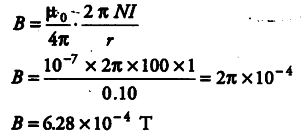# What is the magnitude of the magnetic field at the centre of the coil?

A tightly wound 100 turns coil of radius 10 cm is carrying a current of 1A. What is the magnitude of the magnetic field at the centre of the coil?

Here, N =100, r = 10 cm = 0.10 m, / = 1A
Magnetic field at the centre of coil given by